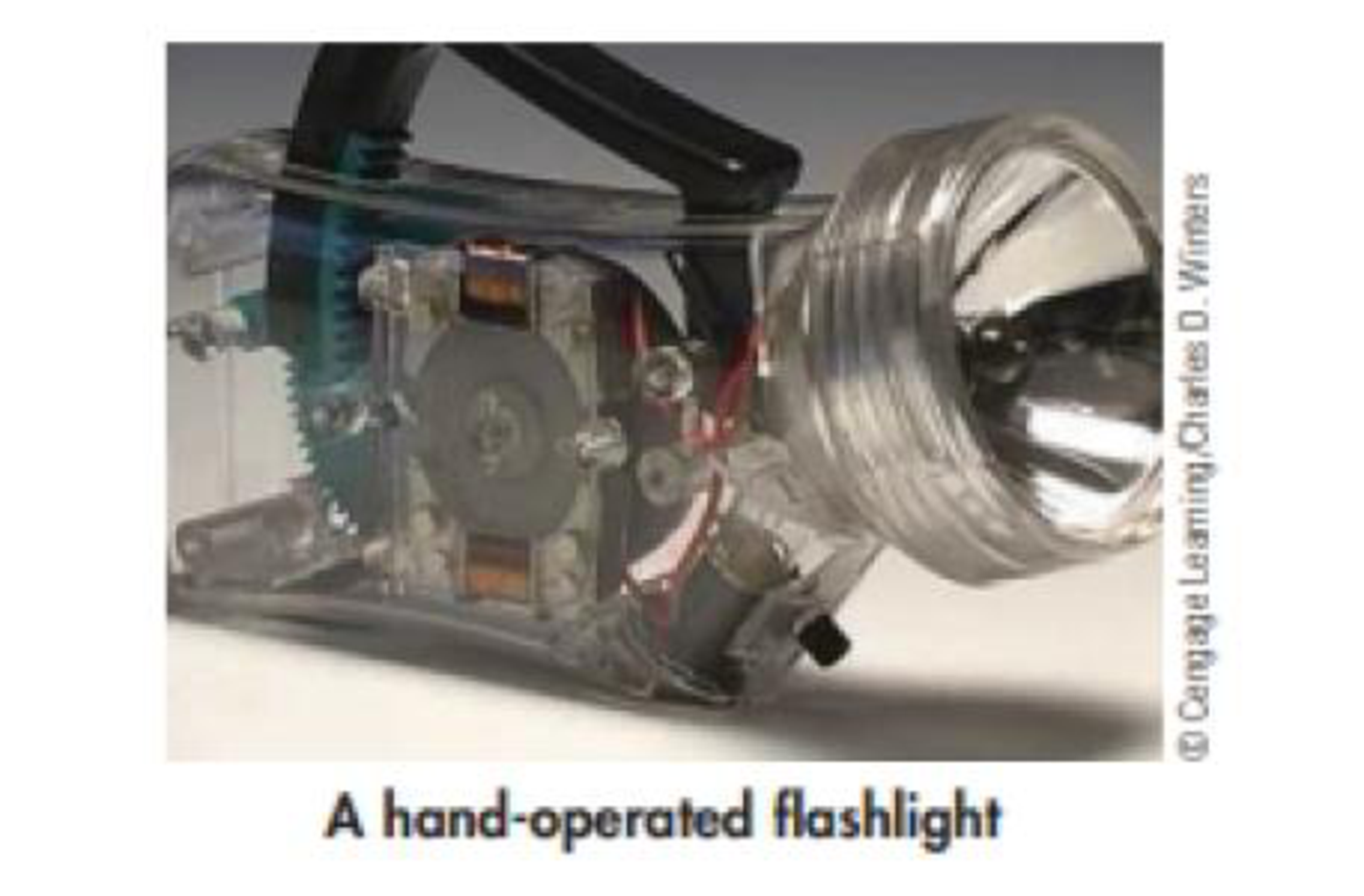Chapter 1, Problem 19PS

Chapter
Section
Textbook Problem

The flashlight in the photo does not use batteries. Instead, you move a lever, which turns a geared mechanism and finally results in light from the bulb. What type of energy is used to move the lever? What type or types of energy are produced?Interpretation Introduction

Interpretation:

The types of energy used to move lever and type of energy produced has to be given.

Concept Introduction:

Energy:

Energy is the capacity to do work, and can neither be created nor destroyed.  Generally, chemical reaction almost always either release or absorb energy.

Types of energy:

Kinetic energy is the energy associated with motion.

Potential energy is the energy results from an object’s position or state.

Explanation

The motion of lever indicates the motion of macroscopic objects by which the energy is supplied by the mechanical work.  Hence the energy used to move the lever is mechanical energy.

The mechanical work results in the light ge...

Still sussing out bartleby?

Check out a sample textbook solution.

See a sample solution

The Solution to Your Study Problems

Bartleby provides explanations to thousands of textbook problems written by our experts, many with advanced degrees!

Get Started

Find more solutions based on key concepts